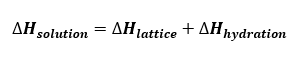# Problem: (a) Use the following data to calculate the combined heat of hydration for the ions in sodium acetate (NaC2H3O2): ΔHlattice = 763 kJ/mol ΔHsoln = 17.3 kJ/mol(b) Which ion contributes more to the answer for part (a)? Why?

###### FREE Expert Solution

To solve this problem, recall the formula that relates the heat of solution, lattice energy and heat of hydration:Given:

ΔHsolution = 17.3 kJ/mol

ΔHlattice = 763 kJ/mol

(a) Solve for ΔHhydration:

86% (116 ratings)###### Problem Details

(a) Use the following data to calculate the combined heat of hydration for the ions in sodium acetate (NaC2H3O2): ΔHlattice = 763 kJ/mol ΔHsoln = 17.3 kJ/mol

(b) Which ion contributes more to the answer for part (a)? Why?